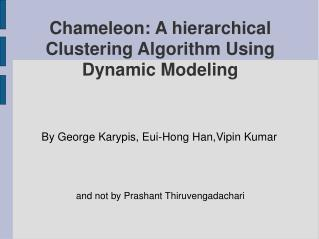DownloadDownload PresentationChameleon: A hierarchical Clustering Algorithm Using Dynamic Modeling

# Chameleon: A hierarchical Clustering Algorithm Using Dynamic Modeling

Download Presentation## Chameleon: A hierarchical Clustering Algorithm Using Dynamic Modeling

- - - - - - - - - - - - - - - - - - - - - - - - - - - E N D - - - - - - - - - - - - - - - - - - - - - - - - - - -
##### Presentation Transcript

1. Chameleon: A hierarchical Clustering Algorithm Using Dynamic Modeling By George Karypis, Eui-Hong Han,Vipin Kumar • and not by Prashant Thiruvengadachari

2. Existing Algorithms • K-means and PAM • Algorithm assigns K-representational points to the clusters and tries to form clusters based on the distance measure.

3. More algorithms • Other algorithm include CURE, ROCK, CLARANS, etc. • CURE takes into account distance between representatives • ROCK takes into account inter-cluster aggregate connectivity.

4. Chameleon • Two-phase approach • Phase -I • Uses a graph partitioning algorithm to divide the data set into a set of individual clusters. • Phase -II • uses an agglomerative hierarchical mining algorithm to merge the clusters.

5. So, basically..

6. Why not stop with Phase-I? We've got the clusters, haven't we ? • Chameleon(Phase-II) takes into account • Inter Connetivity • Relative closeness • Hence, chameleon takes into account features intrinsic to a cluster.

7. Constructing a sparse graph • Using KNN • Data points that are far away are completely avoided by the algorithm (reducing the noise in the dataset)‏ • captures the concept of neighbourhood dynamically by taking into account the density of the region.

8. What do you do with the graph ? • Partition the KNN graph such that the edge cut is minimized. • Reason: Since edge cut represents similarity between the points, less edge cut => less similarity. • Multi-level graph partitioning algorithms to partition the graph – hMeTiS library.

9. Example:

10. Cluster Similarity • Models cluster similarity based on the relative inter-connectivity and relative closeness of the clusters.

11. Relative Inter-Connectivity • Ci and Cj • RIC= AbsoluteIC(Ci,Cj)‏ internal IC(Ci)+internal IC(Cj) / 2 • where AbsoluteIC(Ci,Cj)= sum of weights of edges that connect Ci with Cj. • internalIC(Ci) = weighted sum of edges that partition the cluster into roughly equal parts.

12. Relative Closeness • Absolute closeness normalized with respect to the internal closeness of the two clusters. • Absolute closeness got by average similarity between the points in Ci that are connected to the points in Cj. • average weight of the edges from C(i)->C(j).

13. Internal Closeness…. • Internal closeness of the cluster got by average of the weights of the edges in the cluster.

14. So, which clusters do we merge? • So far, we have got • Relative Inter-Connectivity measure. • Relative Closeness measure. • Using them,

15. Merging the clusters.. • If the relative inter-connectivity measure relative closeness measure are same, choose inter-connectivity. • You can also use, • RI (Ci , C j )≥T(RI) and RC(C i,C j ) ≥ T(RC)‏ • Allows multiple clusters to merge at each level.

16. Good points about the paper : • Nice description of the working of the system. • Gives a note of existing algorithms and as to why chameleon is better. • Not specific to a particular domain.

17. yucky and reasonably yucky parts.. • Not much information given about the Phase-I part of the paper – graph properties ? • Finding the complexity of the algorithm O(nm + n log n + m^2log m)‏ • Different domains require different measures for connectivity and closeness, ...................

18. Questions ?